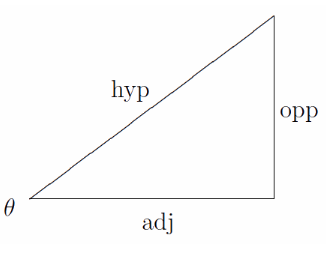# Cos Theta Formula

Amongst all the trigonometric formulas, the most important ones are the right triangle formulas. The Cos θ = Adjacent / Hypotenuse

## Cos angle formula

There are many formulas in trigonometry but there are few most important basic formulas in trigonometry when it comes to a right-angle triangle. The Cos theta or cos θ is the ratio of the adjacent side to the hypotenuse, where θ is one of the acute angles. The cosine formula is as follows:

 $$\begin{array}{l}Cos \Theta = \frac{Adjacent}{Hypotenuse}\end{array}$$## Cos Theta Formula Questions

Example 1:

If Sin x = 4/5, Find the value of Cos x?

Solution: Using Trigonometric identities: Cos2x = 1- Sin2x

Cos2x = 1 – (4/5)2

= 1 – 16/25

= (25 – 16) / 25

= 9/25

Cos x =

$$\begin{array}{l}\sqrt{9/25}\end{array}$$

= 3/5

Example 2: If Sec x = 4/7, find the Cos x?

Solution: As cos x = 1/sec x

Therefore, cos x = 1/ 4/7

= 7/4

## Practice questions

Q1) Find cos x, if sin x =2/5?

Q2) Find Cos a, if Sin a = 3/7?